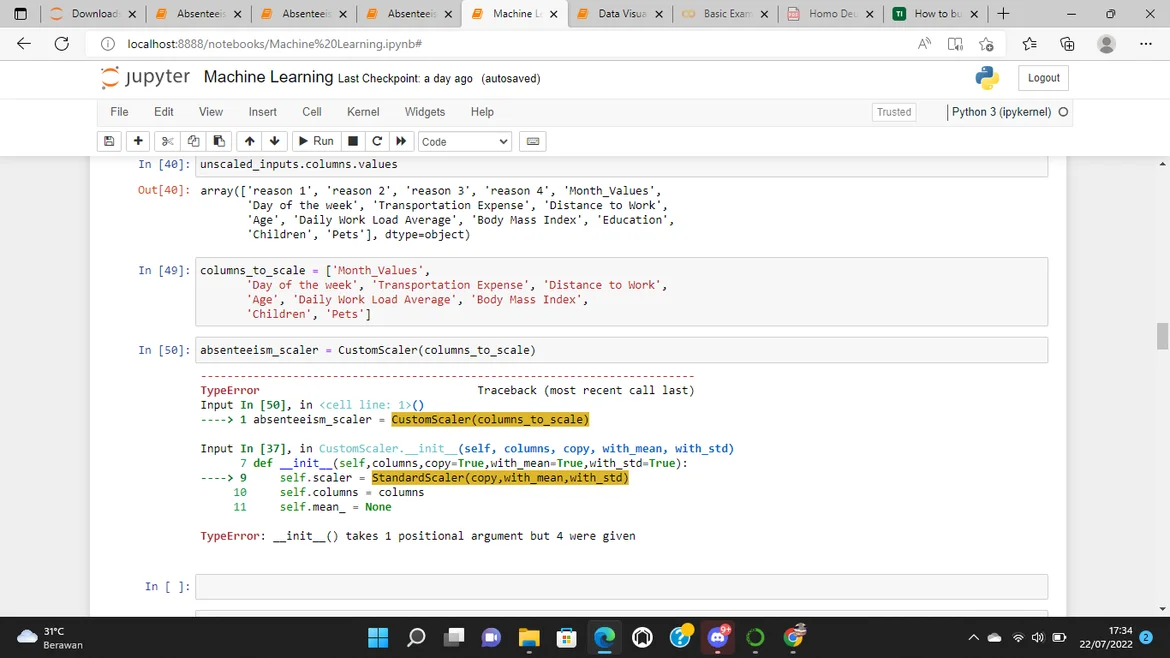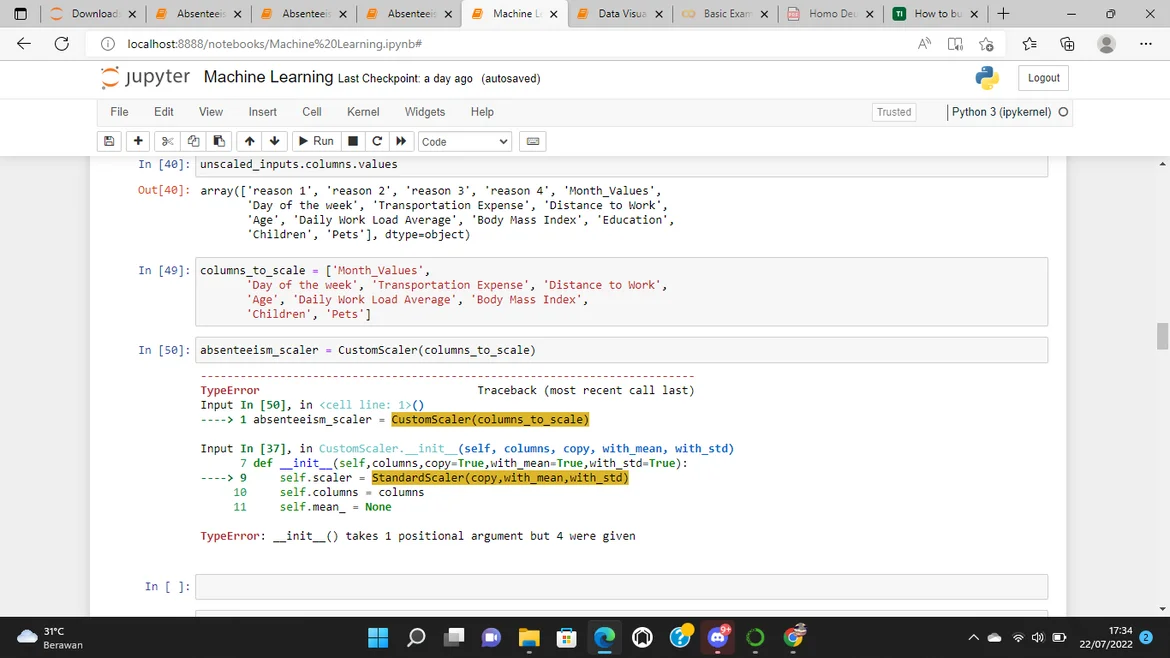23 Jul 2022

Posted on:

22 Jul 2022

0

# Error in JupyterNotebook with sk learn

i have an error while running Standard Scaler. it said that it takes 1 positional argument but 4 were given. when i use your guys answers it siad the same. please enligthen me what to do because i cant continue the course.Posted on:

23 Jul 2022

0

now i got the answer, its from someone in stackoverflow, here's the code

from sklearn.base import BaseEstimator, TransformerMixin
from sklearn.preprocessing import StandardScaler

class CustomScaler(BaseEstimator,TransformerMixin):

def __init__(self,columns,copy=True,with_mean=True,with_std=True):

self.columns = columns
self.copy = copy
self.with_mean = with_mean
self.with_std = with_std

def fit(self, X, y=None):
self.scaler = StandardScaler(copy=self.copy, with_mean=self.with_mean, with_std=self.with_std)
self.scaler.fit(X[self.columns], y)
self.mean_ = np.mean(X[self.columns])
self.var_ = np.var(X[self.columns])
return self

def transform(self, X, y=None, copy=None):

init_col_order = X.columns

X_scaled = pd.DataFrame(self.scaler.transform(X[self.columns]), columns=self.columns)

X_not_scaled = X.loc[:,~X.columns.isin(self.columns)]

return pd.concat([X_not_scaled, X_scaled], axis=1)[init_col_order]

i dont know what happens in the code but it works! i can analyze the data further now, hope it will help someone